Function Repository Resource:

# OrthogonalPolynomialDividedDifference

Evaluate the divided difference of a finite orthogonal polynomial series

Contributed by: Jan Mangaldan
 ResourceFunction["OrthogonalPolynomialDividedDifference"][cof,poly,x,y] evaluates the divided difference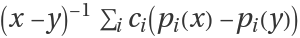, where ci is the (i+1)th element of the list cof.

## Details

The argument poly can be any of the following:
 "ChebyshevFirst" Chebyshev polynomial of the first kind ChebyshevT[i,x] "ChebyshevSecond" Chebyshev polynomial of the second kind ChebyshevU[i,x] "Hermite" Hermite polynomial HermiteH[i,x] "Laguerre" Laguerre polynomial LaguerreL[i,x] "Legendre" Legendre polynomial LegendreP[i,x] {"Gegenbauer",m} Gegenbauer polynomial GegenbauerC[i,m,x] {"Laguerre",a} associated Laguerre polynomial LaguerreL[i,a,x] {"Jacobi",a,b} Jacobi polynomial JacobiP[i,a,b,x]
Strings in specifications like "Legendre" and {"Jacobi",a,b} can be replaced with the corresponding symbols, as in LegendreP and {JacobiP,a,b}.

## Examples

### Basic Examples (2)

Divided difference of a Laguerre series:

 In:=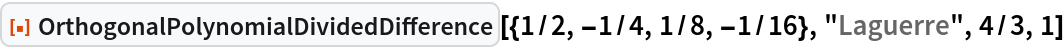Out=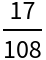Compare with an explicit evaluation using the resource function OrthogonalPolynomialSum:

 In:=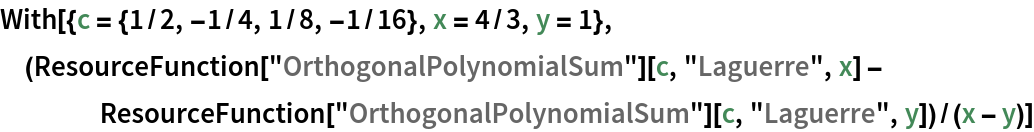Out=### Scope (2)

Divided difference of a Jacobi series with symbolic coefficients, parameters and arguments:

 In:=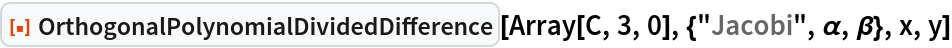Out=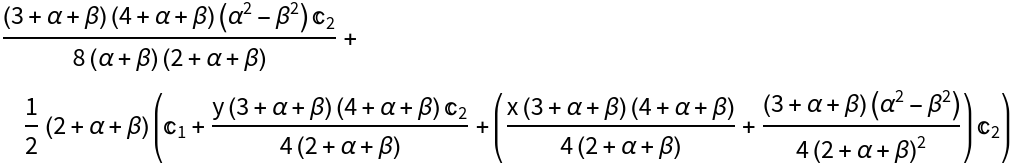An equivalent specification:

 In:=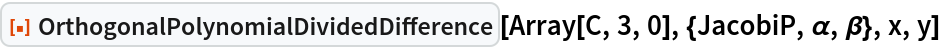Out=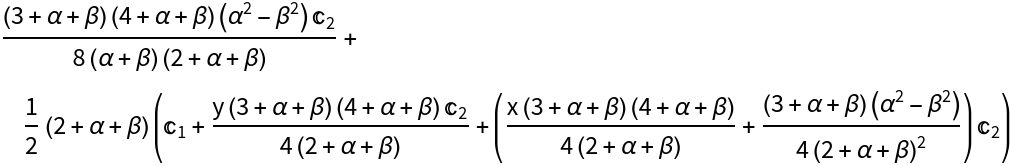### Applications (2)

Directly evaluating the divided difference using the resource function OrthogonalPolynomialSum gives a result that is not very accurate:

 In:=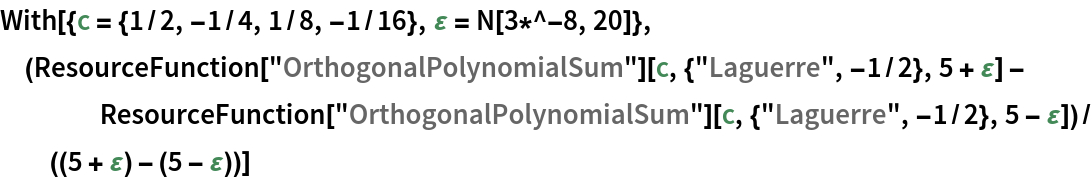Out=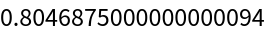OrthogonalPolynomialDividedDifference gives a more accurate result:

 In:=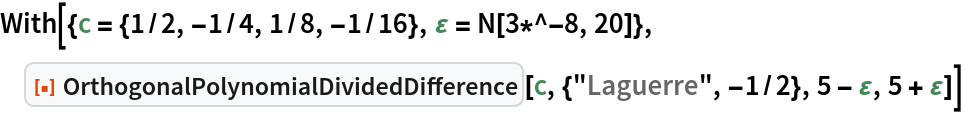Out=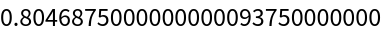Evaluate the q-derivative of a Chebyshev series:

 In:=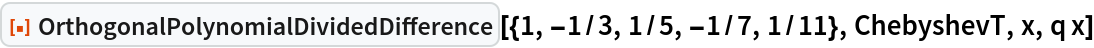Out=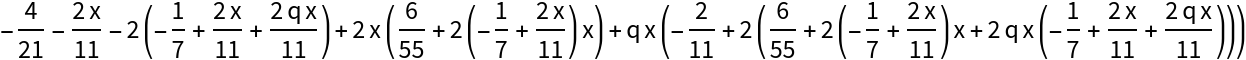In the limit q1, the q-derivative reduces to the derivative:

 In:=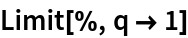Out=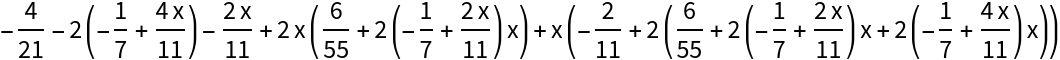In:=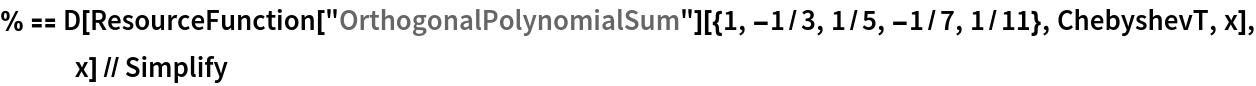Out=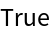### Properties and Relations (2)

OrthogonalPolynomialDividedDifference is symmetric in the arguments x and y:

 In:=Out=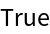If x=y, the result of OrthogonalPolynomialDividedDifference is equal to the derivative of the orthogonal polynomial series, evaluated at x:

 In:=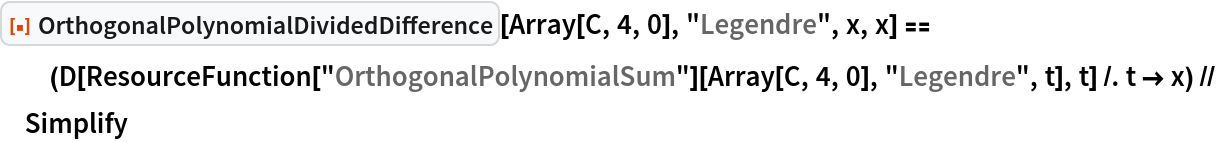Out=## Version History

• 1.0.0 – 06 April 2021